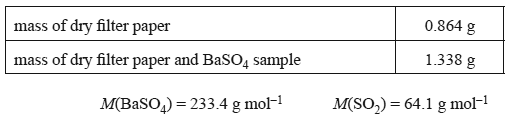Gravimetric analysis(2014 VCE) 1) The conversion of sulfur dioxide to sulfuric acid is used in a number of analytical techniques to determine the amount of analyte present in a substance. The half-equation for this reaction is SO2(aq) + 2H2O(l) => SO42–(aq) + 4H+(aq) + 2e– What type of reaction is this? Solution Sulfur dioxide is often used as a preservative in food and drink. The sulfur dioxide content in dried apricots was determined by gravimetric analysis as follows: • The dried apricots were powderised in a blender. • A sample of the apricot powder weighing 50.00 g was put into a conical fl ask containing 100 mL of de-ionised water. • A 3% solution of hydrogen peroxide was added to convert the dissolved sulfur dioxide to sulfate ions. • An excess of barium chloride solution was then added. The barium sulfate precipitate was fi ltered off, dried and weighed to constant mass. The equation for the precipitation of barium sulfate is Ba2+(aq) + SO42–(aq) => BaSO4(s) The following results were recorded.a) Determine the percentage, by mass, of SO2 in the apricot sample. Solutionb) Express the concentration of sulfur dioxide in the apricot sample in ppm. Solutionc) Why were the apricots ground to a fi ne powder prior to the reaction? Solution2) Oil absorbs a small amount of water from its surroundings. In 1935, Karl Fischer, a German chemist, published a technique for the determination of the water content in oil samples. This technique also involves the conversion of sulfur dioxide to sulfate. In this analytical technique, the reactants – iodine, sulfur dioxide and a base – are all dissolved in methanol. The base is an organic compound and is represented by B in the balanced equation for this reaction. States are not included in this equation. H2O + I2 + SO2 + CH3OH + 3B => [BH]SO4CH3 + 2[BH]I i. What is the mole ratio between iodine and water in this reaction?   Solutionii. The iodine titrating agent was prepared by dissolving 15.0 g of iodine, I2, in methanol using a volumetric flask and making up the volume to 500.0 mL. A 10.0 mL sample of oil was analysed using the iodine solution. The mean titre was found to be 4.95 mL. M(I2) = 253.8 g mol–1 M(H2O) = 18.0 g mol–1 Determine the mass of water present in a 10.0 mL sample of oil. Solutioniii. Determine the percentage by mass of water present if the density of the oil sample is 0.918 g mL–1. Solution